# Potential energy

Potential energyIn the case of a bow and arrow, when the archer does work on the bow, drawing the string back, some of the chemical energy of the archer's body is transformed into elastic potential energy in the bent limbs of the bow. When the string is released, the force between the string and the arrow does work on the arrow. Thus, the potential energy in the bow limbs is transformed into the kinetic energy of the arrow as it takes flight.
Common symbols
PE, U, or V
SI unit joule (J)
Derivations from
other quantities

U = m · g · h (gravitational)
U = ½ · k · x2 (elastic)
U = ½ · C · V2 (electric)

U = -m · B (magnetic)

In physics, potential energy is energy possessed by a body by virtue of its position relative to others, stresses within itself, electric charge, and other factors. Common types include the gravitational potential energy of an object that depends on its mass and its distance from the center of mass of another object, the elastic potential energy of an extended spring, and the electric potential energy of an electric charge in an electric field. The unit for energy in the International System of Units (SI) is the joule, which has the symbol J.

The term potential energy was introduced by the 19th century Scottish engineer and physicist William Rankine, although it has links to Greek philosopher Aristotle's concept of potentiality. Potential energy is associated with forces that act on a body in a way that the total work done by these forces on the body depends only on the initial and final positions of the body in space. These forces, that are called potential forces, can be represented at every point in space by vectors expressed as gradients of a certain scalar function called potential.

Since the work of potential forces acting on a body that moves from a start to an end position is determined only by these two positions, and does not depend on the trajectory of the body, there is a function known as potential or potential energy that can be evaluated at the two positions to determine this work.

## Overview

Potential energy is the stored energy of an object. It is the energy by virtue of an object's position relative to other objects. Potential energy is often associated with restoring forces such as a spring or the force of gravity. The action of stretching the spring or lifting the mass is performed by an external force that works against the force field of the potential. This work is stored in the force field, which is said to be stored as potential energy. If the external force is removed the force field acts on the body to perform the work as it moves the body back to the initial position, reducing the stretch of the spring or causing a body to fall.

Suppose a ball which mass is m, and it is in h position in height. If the acceleration of free fall is g, the weight of the ball is mg. So the total work or energy is force × displacement = mg × h = mgh
Ep = mgh

The more formal definition is that potential energy is the energy difference between the energy of an object in a given position and its energy at a reference position.

There are various types of potential energy, each associated with a particular type of force. For example, the work of an elastic force is called elastic potential energy; work of the gravitational force is called gravitational potential energy; work of the Coulomb force is called electric potential energy; work of the strong nuclear force or weak nuclear force acting on the baryon charge is called nuclear potential energy; work of intermolecular forces is called intermolecular potential energy. Chemical potential energy, such as the energy stored in fossil fuels, is the work of the Coulomb force during rearrangement of mutual positions of electrons and nuclei in atoms and molecules. Thermal energy usually has two components: the kinetic energy of random motions of particles and the potential energy of their mutual positions.

Forces derivable from a potential are also called conservative forces. The work done by a conservative force iswhereis the change in the potential energy associated with the force. The negative sign provides the convention that work done against a force field increases potential energy, while work done by the force field decreases potential energy. Common notations for potential energy are U, V, also Ep.

## Work and potential energy

Potential energy is closely linked with forces. If the work done by a force on a body that moves from A to B does not depend on the path between these points, then the work of this force measured from A assigns a scalar value to every other point in space and defines a scalar potential field. In this case, the force can be defined as the negative of the vector gradient of the potential field.

If the work for an applied force is independent of the path, then the work done by the force is evaluated at the start and end of the trajectory of the point of application. This means that there is a function U (x), called a "potential," that can be evaluated at the two points xA and xB to obtain the work over any trajectory between these two points. It is tradition to define this function with a negative sign so that positive work is a reduction in the potential, that iswhere C is the trajectory taken from A to B. Because the work done is independent of the path taken, then this expression is true for any trajectory, C, from A to B.

The function U(x) is called the potential energy associated with the applied force. Examples of forces that have potential energies are gravity and spring forces.

### Derivable from a potential

In this section the relationship between work and potential energy is presented in more detail. The line integral that defines work along curve C takes a special form if the force F is related to a scalar field φ(x) so thatIn this case, work along the curve is given bywhich can be evaluated using the gradient theorem to obtainThis shows that when forces are derivable from a scalar field, the work of those forces along a curve C is computed by evaluating the scalar field at the start point A and the end point B of the curve. This means the work integral does not depend on the path between A and B and is said to be independent of the path.

Potential energy U=-φ(x) is traditionally defined as the negative of this scalar field so that work by the force field decreases potential energy, that isIn this case, the application of the del operator to the work function yields,and the force F is said to be "derivable from a potential." This also necessarily implies that F must be a conservative vector field. The potential U defines a force F at every point x in space, so the set of forces is called a force field.

### Computing potential energy

Given a force field F(x), evaluation of the work integral using the gradient theorem can be used to find the scalar function associated with potential energy. This is done by introducing a parameterized curve γ(t)=r(t) from γ(a)=A to γ(b)=B, and computing,For the force field F, let v= dr/dt, then the gradient theorem yields,The power applied to a body by a force field is obtained from the gradient of the work, or potential, in the direction of the velocity v of the point of application, that isExamples of work that can be computed from potential functions are gravity and spring forces.

## Potential energy for near Earth gravity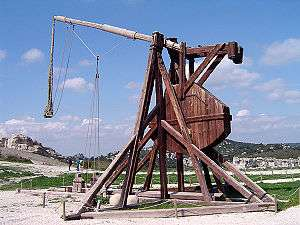A trebuchet uses the gravitational potential energy of the counterweight to throw projectiles over two hundred metres

The following function is called the potential energy of a near earth gravity field:where m is in kg, g is 9.81 for earth and h is in metres.

In classical physics, gravity exerts a constant downward force F=(0, 0, Fz) on the center of mass of a body moving near the surface of the Earth. The work of gravity on a body moving along a trajectory r(t) = (x(t), y(t), z(t)), such as the track of a roller coaster is calculated using its velocity, v=(vx, vy, vz), to obtainwhere the integral of the vertical component of velocity is the vertical distance. Notice that the work of gravity depends only on the vertical movement of the curve r(t).

## Potential energy for a linear springSprings are used for storing elastic potential energy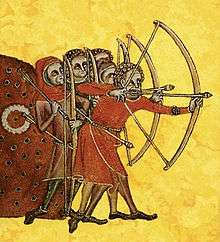Archery is one of humankind's oldest applications of elastic potential energy

A horizontal spring exerts a force F = (−kx, 0, 0) that is proportional to its deflection in the x direction. The work of this spring on a body moving along the space curve s(t) = (x(t), y(t), z(t)), is calculated using its velocity, v = (vx, vy, vz), to obtainFor convenience, consider contact with the spring occurs at t = 0, then the integral of the product of the distance x and the x-velocity, xvx, is x2/2.

The functionis called the potential energy of a linear spring.

Elastic potential energy is the potential energy of an elastic object (for example a bow or a catapult) that is deformed under tension or compression (or stressed in formal terminology). It arises as a consequence of a force that tries to restore the object to its original shape, which is most often the electromagnetic force between the atoms and molecules that constitute the object. If the stretch is released, the energy is transformed into kinetic energy.

## Potential energy for gravitational forces between two bodies

The gravitational potential function, also known as gravitational potential energy, is:The negative sign follows the convention that work is gained from a loss of potential energy.

### Derivation

Gravitational potential energy between two bodies in space is obtained from the force exerted by a mass M on another mass m is given bywhere r is the position vector from the object with mass M to the object with mass m, and G is the gravitational constant.

This can also be expressed aswhereis a vector of length 1 pointing from M to m.

Let the mass m move at the velocity v then the work of gravity on this mass as it moves from position r(t1) to r(t2) is given byNotice that the position and velocity of the mass m are given bywhere er and et are the radial and tangential unit vectors directed relative to the vector from M to m. Use this to simplify the formula for work of gravity to,This calculation uses the fact that## Potential energy for electrostatic forces between two bodies

The electrostatic force exerted by a charge Q on another charge q is given bywhere r is the position vector from Q to q and ε0 is the vacuum permittivity. This may also be written using Coulomb's constant ke = 1 ⁄ 4πε0.

The work W required to move q from A to any point B in the electrostatic force field is given by the potential function## Reference level

The potential energy is a function of the state a system is in, and is defined relative to that for a particular state. This reference state is not always a real state, it may also be a limit, such as with the distances between all bodies tending to infinity, provided that the energy involved in tending to that limit is finite, such as in the case of inverse-square law forces. Any arbitrary reference state could be used, therefore it can be chosen based on convenience.

Typically the potential energy of a system depends on the relative positions of its components only, so the reference state can also be expressed in terms of relative positions.

## Gravitational potential energy

Gravitational energy is the potential energy associated with gravitational force, as work is required to elevate objects against Earth's gravity. The potential energy due to elevated positions is called gravitational potential energy, and is evidenced by water in an elevated reservoir or kept behind a dam. If an object falls from one point to another point inside a gravitational field, the force of gravity will do positive work on the object, and the gravitational potential energy will decrease by the same amount.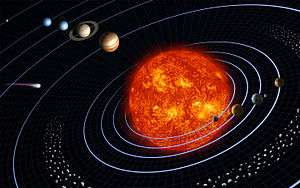Gravitational force keeps the planets in orbit around the Sun

Consider a book placed on top of a table. As the book is raised from the floor, to the table, some external force works against the gravitational force. If the book falls back to the floor, the "falling" energy the book receives is provided by the gravitational force. Thus, if the book falls off the table, this potential energy goes to accelerate the mass of the book and is converted into kinetic energy. When the book hits the floor this kinetic energy is converted into heat, deformation and sound by the impact.

The factors that affect an object's gravitational potential energy are its height relative to some reference point, its mass, and the strength of the gravitational field it is in. Thus, a book lying on a table has less gravitational potential energy than the same book on top of a taller cupboard, and less gravitational potential energy than a heavier book lying on the same table. An object at a certain height above the Moon's surface has less gravitational potential energy than at the same height above the Earth's surface because the Moon's gravity is weaker. Note that "height" in the common sense of the term cannot be used for gravitational potential energy calculations when gravity is not assumed to be a constant. The following sections provide more detail.

### Local approximation

The strength of a gravitational field varies with location. However, when the change of distance is small in relation to the distances from the center of the source of the gravitational field, this variation in field strength is negligible and we can assume that the force of gravity on a particular object is constant. Near the surface of the Earth, for example, we assume that the acceleration due to gravity is a constant g = 9.8 m/s2 ("standard gravity"). In this case, a simple expression for gravitational potential energy can be derived using the W = Fd equation for work, and the equationThe amount of gravitational potential energy possessed by an elevated object is equal to the work done against gravity in lifting it. The work done equals the force required to move it upward multiplied with the vertical distance it is moved (remember W = Fd). The upward force required while moving at a constant velocity is equal to the weight, mg, of an object, so the work done in lifting it through a height h is the product mgh. Thus, when accounting only for mass, gravity, and altitude, the equation is:where U is the potential energy of the object relative to its being on the Earth's surface, m is the mass of the object, g is the acceleration due to gravity, and h is the altitude of the object. If m is expressed in kilograms, g in m/s2 and h in metres then U will be calculated in joules.

Hence, the potential difference is### General formula

However, over large variations in distance, the approximation that g is constant is no longer valid, and we have to use calculus and the general mathematical definition of work to determine gravitational potential energy. For the computation of the potential energy we can integrate the gravitational force, whose magnitude is given by Newton's law of gravitation, with respect to the distance r between the two bodies. Using that definition, the gravitational potential energy of a system of masses m1 and M2 at a distance r using gravitational constant G is,

where K is an arbitrary constant dependent on the choice of datum from which potential is measured. Choosing the convention that K=0 (i.e. in relation to a point at infinity) makes calculations simpler, albeit at the cost of making U negative; for why this is physically reasonable, see below.

Given this formula for U, the total potential energy of a system of n bodies is found by summing, for allpairs of two bodies, the potential energy of the system of those two bodies.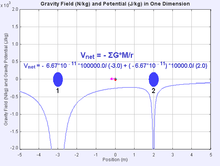Gravitational potential summationConsidering the system of bodies as the combined set of small particles the bodies consist of, and applying the previous on the particle level we get the negative gravitational binding energy. This potential energy is more strongly negative than the total potential energy of the system of bodies as such since it also includes the negative gravitational binding energy of each body. The potential energy of the system of bodies as such is the negative of the energy needed to separate the bodies from each other to infinity, while the gravitational binding energy is the energy needed to separate all particles from each other to infinity.therefore,,

### Why choose a convention where gravitational energy is negative?

As with all potential energies, only differences in gravitational potential energy matter for most physical purposes, and the choice of zero point is arbitrary. Given that there is no reasonable criterion for preferring one particular finite r over another, there seem to be only two reasonable choices for the distance at which U becomes zero:and. The choice ofat infinity may seem peculiar, and the consequence that gravitational energy is always negative may seem counterintuitive, but this choice allows gravitational potential energy values to be finite, albeit negative.

The singularity atin the formula for gravitational potential energy means that the only other apparently reasonable alternative choice of convention, withfor, would result in potential energy being positive, but infinitely large for all nonzero values of r, and would make calculations involving sums or differences of potential energies beyond what is possible with the real number system. Since physicists abhor infinities in their calculations, and r is always non-zero in practice, the choice ofat infinity is by far the more preferable choice, even if the idea of negative energy in a gravity well appears to be peculiar at first.

The negative value for gravitational energy also has deeper implications that make it seem more reasonable in cosmological calculations where the total energy of the universe can meaningfully be considered; see inflation theory for more on this.

### Uses

Gravitational potential energy has a number of practical uses, notably the generation of pumped-storage hydroelectricity. For example, in Dinorwig, Wales, there are two lakes, one at a higher elevation than the other. At times when surplus electricity is not required (and so is comparatively cheap), water is pumped up to the higher lake, thus converting the electrical energy (running the pump) to gravitational potential energy. At times of peak demand for electricity, the water flows back down through electrical generator turbines, converting the potential energy into kinetic energy and then back into electricity. The process is not completely efficient and some of the original energy from the surplus electricity is in fact lost to friction.

Gravitational potential energy is also used to power clocks in which falling weights operate the mechanism.

It's also used by counterweights for lifting up an elevator, crane, or sash window.

Roller coasters are an entertaining way to utilize potential energy - chains are used to move a car up an incline (building up gravitational potential energy), to then have that energy converted into kinetic energy as it falls.

Another practical use is utilizing gravitational potential energy to descend (perhaps coast) downhill in transportation such as the descent of an automobile, truck, railroad train, bicycle, airplane, or fluid in a pipeline. In some cases the kinetic energy obtained from potential energy of descent may be used to start ascending the next grade such as what happens when a road is undulating and has frequent dips. The commercialization of stored energy (in the form of rail cars raised to higher elevations) that is then converted to electrical energy when needed by an electrical grid, is being undertaken in the United States in a system called Advanced Rail Energy Storage (ARES).

Further information: Gravitational potential energy storage

## Chemical potential energy

Main article: Chemical energy

Chemical potential energy is a form of potential energy related to the structural arrangement of atoms or molecules. This arrangement may be the result of chemical bonds within a molecule or otherwise. Chemical energy of a chemical substance can be transformed to other forms of energy by a chemical reaction. As an example, when a fuel is burned the chemical energy is converted to heat, same is the case with digestion of food metabolized in a biological organism. Green plants transform solar energy to chemical energy through the process known as photosynthesis, and electrical energy can be converted to chemical energy through electrochemical reactions.

The similar term chemical potential is used to indicate the potential of a substance to undergo a change of configuration, be it in the form of a chemical reaction, spatial transport, particle exchange with a reservoir, etc.

## Electric potential energy

An object can have potential energy by virtue of its electric charge and several forces related to their presence. There are two main types of this kind of potential energy: electrostatic potential energy, electrodynamic potential energy (also sometimes called magnetic potential energy).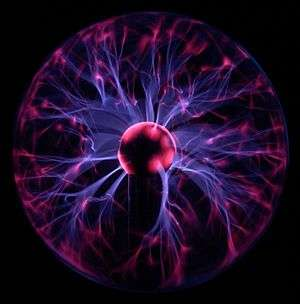Plasma formed inside a gas filled sphere

### Electrostatic potential energy

Electrostatic potential energy between two bodies in space is obtained from the force exerted by a charge Q on another charge q which is given bywhere r is the position vector from Q to q and ε0 is the vacuum permittivity. This may also be written using Coulomb's constant ke = 1 ⁄ 4πε0.

If the electric charge of an object can be assumed to be at rest, then it has potential energy due to its position relative to other charged objects. The electrostatic potential energy is the energy of an electrically charged particle (at rest) in an electric field. It is defined as the work that must be done to move it from an infinite distance away to its present location, adjusted for non-electrical forces on the object. This energy will generally be non-zero if there is another electrically charged object nearby.

The work W required to move q from A to any point B in the electrostatic force field is given by the potential functionA related quantity called electric potential (commonly denoted with a V for voltage) is equal to the electric potential energy per unit charge.

### Magnetic potential energy

The energy of a magnetic moment m in an externally produced magnetic B-field B has potential energyThe magnetization M in a field iswhere the integral can be over all space or, equivalently, where M is nonzero. Magnetic potential energy is the form of energy related not only to the distance between magnetic materials, but also to the orientation, or alignment, of those materials within the field. For example, the needle of a compass has the lowest magnetic potential energy when it is aligned with the north and south poles of the Earth's magnetic field. If the needle is moved by an outside force, torque is exerted on the magnetic dipole of the needle by the Earth's magnetic field, causing it to move back into alignment. The magnetic potential energy of the needle is highest when its field is in the same direction as the Earth's magnetic field. Two magnets will have potential energy in relation to each other and the distance between them, but this also depends on their orientation. If the opposite poles are held apart, the potential energy will be the highest when they are near the edge of their attraction, and the lowest when they pull together. Conversely, like poles will have the highest potential energy when forced together, and the lowest when they spring apart.

## Nuclear potential energy

Nuclear potential energy is the potential energy of the particles inside an atomic nucleus. The nuclear particles are bound together by the strong nuclear force. Weak nuclear forces provide the potential energy for certain kinds of radioactive decay, such as beta decay.

Nuclear particles like protons and neutrons are not destroyed in fission and fusion processes, but collections of them have less mass than if they were individually free, and this mass difference is liberated as heat and radiation in nuclear reactions (the heat and radiation have the missing mass, but it often escapes from the system, where it is not measured). The energy from the Sun is an example of this form of energy conversion. In the Sun, the process of hydrogen fusion converts about 4 million tonnes of solar matter per second into electromagnetic energy, which is radiated into space.

## Forces and potential energy

Potential energy is closely linked with forces. If the work done by a force on a body that moves from A to B does not depend on the path between these points, then the work of this force measured from A assigns a scalar value to every other point in space and defines a scalar potential field. In this case, the force can be defined as the negative of the vector gradient of the potential field.

For example, gravity is a conservative force. The associated potential is the gravitational potential, often denoted byor, corresponding to the energy per unit mass as a function of position. The gravitational potential energy of two particles of mass M and m separated by a distance r isThe gravitational potential (specific energy) of the two bodies iswhereis the reduced mass.

The work done against gravity by moving an infinitesimal mass from point A withto point B withisand the work done going back the other way isso that the total work done in moving from A to B and returning to A isIf the potential is redefined at A to beand the potential at B to be, whereis a constant (i.e.can be any number, positive or negative, but it must be the same at A as it is at B) then the work done going from A to B isas before.

In practical terms, this means that one can set the zero ofandanywhere one likes. One may set it to be zero at the surface of the Earth, or may find it more convenient to set zero at infinity (as in the expressions given earlier in this section).

A conservative force can be expressed in the language of differential geometry as a closed form. As Euclidean space is contractible, its de Rham cohomology vanishes, so every closed form is also an exact form, and can be expressed as the gradient of a scalar field. This gives a mathematical justification of the fact that all conservative forces are gradients of a potential field.

## Notes

1. Jain, Mahesh C. "Fundamental forces and laws: a brief review". Textbook Of Engineering Physics, Part 1. PHI Learning Pvt. Ltd. p. 10. ISBN 9788120338623.
2. McCall, Robert P. (2010). "Energy, Work and Metabolism". Physics of the Human Body. JHU Press. p. 74. ISBN 978-0-8018-9455-8.
3. William John Macquorn Rankine (1853) "On the general law of the transformation of energy," Proceedings of the Philosophical Society of Glasgow, vol. 3, no. 5, pages 276-280; reprinted in: (1) Philosophical Magazine, series 4, vol. 5, no. 30, pages 106-117 (February 1853); and (2) W. J. Millar, ed., Miscellaneous Scientific Papers: by W. J. Macquorn Rankine, ... (London, England: Charles Griffin and Co., 1881), part II, pages 203-208.
4. Smith, Crosbie (1998). The Science of Energy - a Cultural History of Energy Physics in Victorian Britain. The University of Chicago Press. ISBN 0-226-76420-6.
5. Brown, Theodore L. (2006). Chemistry The Central Science. Upper Saddle River, New Jersey: Pearson Education, Inc. p. 168. ISBN 0-13-109686-9.
6. John Robert Taylor (2005). Classical Mechanics. University Science Books. ISBN 978-1-891389-22-1. Retrieved 30 July 2013.
7. Burton Paul (1979). Kinematics and dynamics of planar machinery. Prentice-Hall. ISBN 978-0-13-516062-6. Retrieved 30 July 2013.
8. Feynman, Richard P. (2011). "Work and potential energy". The Feynman Lectures on Physics, Vol. I. Basic Books. p. 13. ISBN 978-0-465-02493-3.
9. "Energy storage - Packing some power". The Economist. 2011-03-03. Retrieved 2012-03-11.
10. Jacob, Thierry.Pumped storage in Switzerland - an outlook beyond 2000 Stucky. Accessed: 13 February 2012.
11. Levine, Jonah G. Pumped Hydroelectric Energy Storage and Spatial Diversity of Wind Resources as Methods of Improving Utilization of Renewable Energy Sources page 6, University of Colorado, December 2007. Accessed: 12 February 2012.
12. Yang, Chi-Jen. Pumped Hydroelectric Storage Duke University. Accessed: 12 February 2012.
13. Energy Storage Hawaiian Electric Company. Accessed: 13 February 2012.
14. Downing, Louise. Ski Lifts Help Open \$25 Billion Market for Storing Power, Bloomberg News online, 6 September 2012
15. Kernan, Aedan. Storing Energy on Rail Tracks, Leonardo-Energy.org website, 30 October 2013
16. Aharoni, Amikam (1996). Introduction to the theory of ferromagnetism (Repr. ed.). Oxford: Clarendon Pr. ISBN 0-19-851791-2.
17. Jackson, John David (1975). Classical electrodynamics (2d ed.). New York: Wiley. ISBN 0-471-43132-X.
18. Livingston, James D. (2011). Rising Force: The Magic of Magnetic Levitation. President and Fellows of Harvard College. p. 152.
19. Kumar, Narinder (2004). Comprehensive Physics XII. Laxmi Publications. p. 713.

## References

• Serway, Raymond A.; Jewett, John W. (2010). Physics for Scientists and Engineers (8th ed.). Brooks/Cole cengage. ISBN 1-4390-4844-4.
• Tipler, Paul (2004). Physics for Scientists and Engineers: Mechanics, Oscillations and Waves, Thermodynamics (5th ed.). W. H. Freeman. ISBN 0-7167-0809-4.